## Closed Loop Gain of Non Inverting Amplifier:

The circuit shown in Fig. 14.7 is commonly known as a Non-Inverting amplifier with feedback (or closed loop Gain Non-inverting amplifier), because it used a feedback and the input signal is applied to the non-inverting input terminal of the opamp.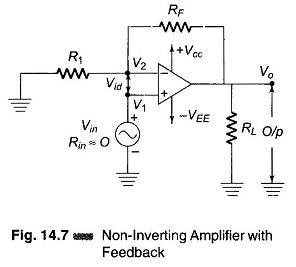The closed loop gain AF = Vo/Vin

From the circuit (Fig. 14.7), the output voltage is given by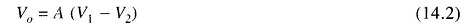But V1 = Vin and V2 = VF, VF can be found by using the voltage divider law.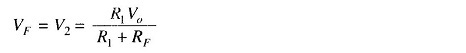Substituting values of V1 and V2 Eq. (14.2) we have,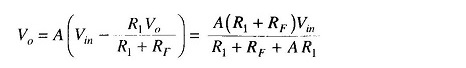Generally A is very large typically 105 hence A R1 > (R1 + RF)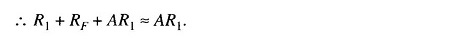Therefore,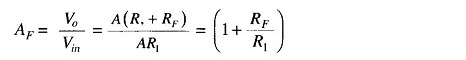Therefore, the closed loop voltage gain for an non-inverting amplifier is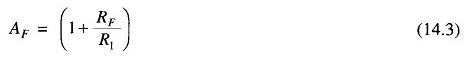Scroll to Top